# Left handed

It is known that 25% of the population is left-handed. What is the probability that there is a maximum of three left-handers at a seminar where there are 30 participants?

Result

p =  3.745 %

#### Solution:Leave us a comment of example and its solution (i.e. if it is still somewhat unclear...):Be the first to comment!#### To solve this verbal math problem are needed these knowledge from mathematics:

Looking for a statistical calculator? Our percentage calculator will help you quickly calculate various typical tasks with percentages. Would you like to compute count of combinations?

## Next similar examples:

1. Lottery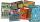Fernando has two lottery tickets each from other lottery. In the first is 973 000 lottery tickets from them wins 687 000, the second has 1425 000 lottery tickets from them wins 1425 000 tickets. What is the probability that at least one Fernando's ticket w
2. CardsSuppose that are three cards in the hats. One is red on both sides, one of which is black on both sides, and a third one side red and the second black. We are pulled out of a hat randomly one card and we see that one side of it is red. What is the probabi
3. Class - boys and girls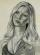In the class are 60% boys and 40% girls. Long hair has 10% boys and 80% girls. a) What is the probability that a randomly chosen person has long hair? b) The selected person has long hair. What is the probability that it is a girl?
4. Probability of intersectionThree students have a probability of 0.7,0.5 and 0.4 to graduated from university respectively. What is the probability that at least one of them will be graduated?
5. Win in raffle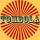The raffle tickets were sold 200, 5 of which were winning. What is the probability that Peter, who bought one ticket will win?
6. Medicine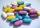We test medicine on 6 patients. For all drug doesn't work. If the drug success rate of 20%, what is the probability that medicine does not work?
7. TestThe teacher prepared a test with ten questions. The student has the option to choose one correct answer from the four (A, B, C, D). The student did not get a written exam at all. What is the probability that: a) He answers half correctly. b) He answers.
8. Probability of malaria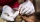A survey carried out at a certain hospital indicates that the probability that a patient tested positive for malaria is 0.6. What is the probability that two patients selected at random (i) one is negative while the other tested positive? (i) both patien
9. Green - Red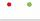We have 5 bags. Each consist one green and 2 red balls. From each we pull just one ball. What is the probability that we doesn't pull any green ball?
10. Family94 boys are born per 100 girls. Determine the probability that there are two boys in a randomly selected family with three children.
11. Component fail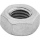There is a 90 percent chance that a particular type of component will perform adequately under high temperature conditions. If the device involved has four such components, determine the probability that the device is inoperable because exactly one of the.
12. Internet anywhere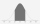In school, 60% of pupils have access to the internet at home. A group of 8 students is chosen at random. Find the probability that a) exactly 5 have access to the internet. b) At least 6 students have access to the internet
13. Utopia IslandA probability of disease A on the island of Utopia is 40%. A probability of occurrence among the men of this island, which make up 60% of all the population (the rest are women), is 50%. What is the probability of occurrence of A disease among women on Uto
14. Three shooters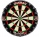Three shooters shoot, each one time, on the same target. The first hit the target with a probability of 0.7; second with a probability of 0.8 and a third with a probability of 0.9. What is the probability to hit the target: a) just once b) at least once c
15. Theorem proveWe want to prove the sentence: If the natural number n is divisible by six, then n is divisible by three. From what assumption we started?
16. ShootersIn army regiment are six shooters. The first shooter target hit with a probability of 49%, next with 75%, 41%, 20%, 34%, 63%. Calculate the probability of target hit when shooting all at once.
17. Probabilities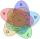If probabilities of A, B and A ∩ B are P (A) = 0.62 P (B) = 0.78 and P (A ∩ B) = 0.26 calculate the following probability (of union. intersect and opposite and its combinations):